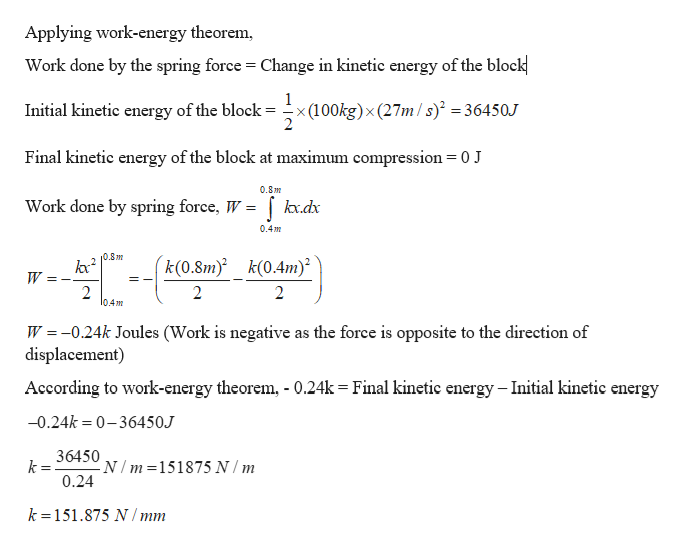Question
1 views
 An initially 'compressed' spring (x1 = 0.4m) is observed to compress an additional 0.4 m before stopping and reversing the 26 m/s horizontal velocity of a 100 kg mass. Determine the spring constant in N/mm.
check_circle

Step 1

Given:

Initial compression in the spring = 0.4 m

Additional compression in the spring = 0.4 m

Final compression in the spring = 0.8 m

Velocity of the block = 26 m/s

Mass of the block = 100 kg

Step 2

Calculating the spring cons...help_outlineImage TranscriptioncloseApplying work-energy theorem Work done by the spring force Change in kinetic energy of the block Initial kinetic energy of the block - x(100kg)x (27m / s) 36450J Final kinetic energy of the block at maximum compression = 0 J 0.8m Work done by spring force, W kx.dx 0.4m 0.8m k(0.8m) k(0.4m) W 2 2 l0.4m W 0.24k Joules (Work is negative as the force is oppo site to the direction of displacement) According to work-energy theorem 0.24k Final kinetic energy - Initial kinetic energy -0.24k 0-36450J 36450 N/m 151875 N/ m 0.24 k=151.875 N/mm fullscreen

### Want to see the full answer?

See Solution

#### Want to see this answer and more?

Solutions are written by subject experts who are available 24/7. Questions are typically answered within 1 hour.*

See Solution
*Response times may vary by subject and question.
Tagged in

### Other